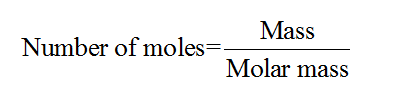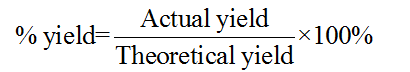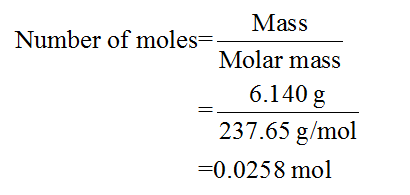# Is my work correct?Question: What is the percent yeild of the nicke(II) coordination?[Ni(H2O)6]Cl2 used = 6.140 g[Ni(en)3]Cl2 produced = 7.001 gWorkmol of [Ni(H2O)6]Cl26.140 g/237.67 g/mol = 0.0258 mol [Ni(H2O)6]Cl2mol of [Ni(en)3]Cl20.0258 mol [Ni(H2O)6]Cl2 x (1 mol [Ni(H2O)6]Cl2/1mol [Ni(en)3]Cl2) = 0.0258 molgrams of [Ni(en)3]Cl20.0258 mol x 309.89 g/mol = 8.00 g% yield 7.001 g/8.00 g = 87.5%

Question
7 views

Is my work correct?

Question: What is the percent yeild of the nicke(II) coordination?

• [Ni(H2O)6]Cl2 used = 6.140 g
• [Ni(en)3]Cl2 produced = 7.001 g

Work

• mol of [Ni(H2O)6]Cl2
• 6.140 g/237.67 g/mol = 0.0258 mol [Ni(H2O)6]Cl2
• mol of [Ni(en)3]Cl2
• 0.0258 mol [Ni(H2O)6]Cl2 x (1 mol [Ni(H2O)6]Cl2/1mol [Ni(en)3]Cl2) = 0.0258 mol
• grams of [Ni(en)3]Cl2
• 0.0258 mol x 309.89 g/mol = 8.00 g
• % yield
• 7.001 g/8.00 g = 87.5%
check_circle

Step 1

The number of moles for a given chemical species refers to the ratio of mass of the species to that of its molar mass. The formula is shown below:Step 2

The percent yield in chemistry can be defined as the ratio of actual yield to the theoretical yield multiplied by 100%. The formula is as follows:Step 3

In the given problem, the mass of [Ni(H2O)6]Cl2 which has been used is given to be 6.140 g.

The molar  mass of [Ni(H2O)6]Cl2 is 237.65 g/mol.

The number of moles of [Ni(H2O)6]Cl2 can be calculated as follows:...

### Want to see the full answer?

See Solution

#### Want to see this answer and more?

Solutions are written by subject experts who are available 24/7. Questions are typically answered within 1 hour.*

See Solution
*Response times may vary by subject and question.
Tagged in

### Chemistry